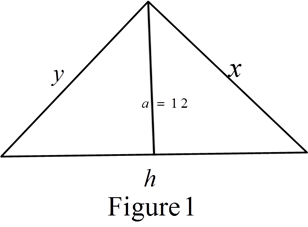# To express: The length of the hypotenuse as a function of the perimeter.### Single Variable Calculus: Concepts...

4th Edition
James Stewart
Publisher: Cengage Learning
ISBN: 9781337687805### Single Variable Calculus: Concepts...

4th Edition
James Stewart
Publisher: Cengage Learning
ISBN: 9781337687805

#### Solutions

Chapter 1, Problem 2P
To determine

## To express: The length of the hypotenuse as a function of the perimeter.

Expert Solution

Solution:

The length of the hypotenuse is h=P22P+24.

### Explanation of Solution

Given:

The altitude perpendicular to the hypotenuse a=12 cm

Formula used:

Area of the triangleA=12(xy) (1)

Pythagoras theorem x2+y2=h2 (2)

Where, h is a hypotenuse, and x, y is the sides of triangle

Perimeter of the triangle P=x+y+h (3)

(x+y)2=x2+y2+2xy

Calculation:

Consider the triangle as shown below in the Figure 1.From the Figure 1, A=12(ah)

Substitute a=12 in above equation,

A12(12h)=6h

From the equation (1),

A= 12(xy)

This implies xy=2A (4)

Consider the equation (x+y)2=x2+y2+2xy

Substitute the values of equation (1) and equation (2) in above equation,

(x+y)2=h2+4A (5)

From equation (3), x+y=Ph

Substitute the value of x+y in equation (4),

(Ph)2=h2+4AP2+h22Ph=h2+4A2Ph=P24Ah=P24A2P

Substitute A=6h in above equation,

h=P24(6h)2P2Ph=P224h2Ph+24h=P2h(2P+24)=P2

On further simplification, the value of h=P22P+24

Therefore, the length of the hypotenuse is h=P22P+24.

### Have a homework question?

Subscribe to bartleby learn! Ask subject matter experts 30 homework questions each month. Plus, you’ll have access to millions of step-by-step textbook answers!